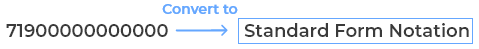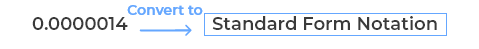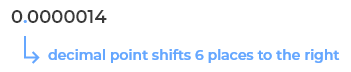Uh Oh! It seems you’re using an Ad blocker!

Since we’ve struggled a lot to makes online calculations for you, we are appealing to you to grant us by disabling the Ad blocker for this domain.# Standard Form Calculator

Convert to Standard Form

Turn a number into a Standard Form, Scientific E-Notation, Engineering Notation, and Real Number format.

Enter a Number
Table of Content
 1 Example of Standard Form 2 How To Write In Standard Form? 3 Real-Life Example of Standard Form 4 What is a million in standard form? 5 How to write 0.00527 in standard form equation? 6 How do you write 200000 in standard form? 7 How do you find the standard form of a graph? 8 What is the standard form of rational number? 9 What is the standard form of a fraction? 10 Why do we use standard form? 11 What is standard form and general form? 12 What is the standard form of 450?
Get The Widget!

Add Standard Form Calculator to your website through which the user of the website will get the ease of utilizing calculator directly. And, this gadget is 100% free and simple to use; additionally, you can add it on multiple online platforms.

Available on App

An online standard form calculator is the tool that allows you to convert the number in the standard form. All you need to enter any number and convert/transform it into standard form (i:e is a number and a power of $$10$$ ). Also, this simple standard form to ordinary calculator allows you to write standard form equation into its ordinary form. You can be able to convert general/integer/decimal/or ordinary form to standard form or vice versa with the help of standard notation converter. The standard form solver works best for students of GCSE Math’s or Science.

Well, in this post you also come to know how to write a number/equation in the standard form calculator online or manually and much more. So, let’s begin with the standard form definition!

Note: People often confused with the terms of standard form and quadratic equation while performing calculations. So, in such a case, use this quadratic equation calculator by calculator-online that helps you to solve a quadratic equation and provides you the accurate results.

So, come to the point (Standard Form)!

## What Is Standard Form In Math?

Well, any number that you can write as a decimal number, between $$1.0$$ and $$10.0$$, and multiplied by a power of $$10$$ is known as the standard form. In other words, it is a way of writing down very large/very small numbers easily. No doubt, it is difficult to read numbers like $$675678888000$$ or $$0.000012345675$$, for the ease you can write it in the form of power of $$10$$. An online standard form converter helps you to convert the numbers into standard form by placing the decimal value in the given number.

Also, you can try this calculator for scientific notation to perform basic math operations on scientific notation & to convert a number to scientific notation.

### Example of Standard Form:

A number is $$600000$$

So, the number is in the standard form written as $$6 ×10^5$$

For better understanding, look at the given table:

 12345 1.2345 x 10^ 4 5.0043e+06 5.0043 x 10^ 6 45 4.5 x 10^ 1 1e-05 0.1 x 10^ -4 0.0003012 0.3012 x 10^ -3 0.00049 0.49 x 10^ -3 3.2e+06 3.2 x 10^ 6 0.00147 0.147 x 10^ -2 23500 2.35 x 10^ 4 80000 8 x 10^ 4 0.4184 0.4184 x 10^ 0 1.496e+08 1.496 x 10^ 8 2.2794e+08 2.2794 x 10^ 8 1.416e+08 1.416 x 10^ 8 9.29e+07 9.29 x 10^ 7 8.603e+07 8.603 x 10^ 7

## Standard Form Calculator By Calculator-Online:

The standard form calculator is a free online tool that simply converts the given number to standard form quickly. There is no need to writing numbers with a power of $$10$$ manually as this standard notation calculator does all for you. Just enter a number to convert it into a standard form, scientific E notation, engineering notation, and real numbers format.

Swipe down to know how this smart standard form generator works!

### How to Convert a Number Into Standard Form With This Standard Notation Calculator:

Don’t fret, this handy calculator helps to write equation in standard form, this tool is 100% free and makes calculations within a fraction of seconds. Just stick to these steps and turn your number into standard form.

Inputs:

• All you need to enter the number that you want to convert into standard form
• Very next, just hit the calculate button

Note: Remember that you can enter number in either integer form or the decimal form, the calculator provides you with the precise results.

Outputs:

The standard notation calculator will show:

• Standard Form for a given number (that is a number and a power of $$10$$)
• Scientific E-Notation
• Engineering Notation
• Real Number

## How To Write In Standard Form?

This is the standard form equation that helps you in writing a number in a standard form, even our calculator also uses the same equation.

$$a = b \times 10^n$$

Let’s take a look!

A number is $$71900000000000$$ – convert to standard notation:• Then, you ought to add a decimal point after it $$(7.)$$• Very, you have to count the number of digits after $$7$$ – you can see there are $$13$$ digits• So, $$71 900 000 000 000$$ is $$7.19 ×10^{13}$$You can also try our free standard form converter calculator to express a number in standard form.

A number is $$0.000 0014$$:• First of all, you have to write the very first non-zero digit – here you can see it is $$(1)$$• Very next, you have to add a decimal point after it: $$(1.)$$• Remember that the decimal point shifts $$6$$ places to the right• So, it represent as $$1.4 ×10^{-6}$$Well, there is no need to stick on such as lengthy calculations, you just have to enter the values into the designated field of the above standard form math calculator, and the tool will take care rest!

## Real-Life Example of Standard Form:

As the distance between the Sun and Mars is $$141,700,000 \text{ miles}$$ or $$228,000,000 \text{ km}$$, how we can write the distance in the standard form!

Convert to standard form:

It can be written as $$1.417×10^8 \text {miles}$$ or $$2.28×10^8 \text {km}$$

You can try the standard notation converter to write the distance in the standard form.

You can notice that astronomers, biologist, engineers, physicists, and many others encounter quantities whose measures involves very small or very large numbers. For better understanding, let’s find the example:

The distance of the earth from the sun is referred to as approximately 144,000,000,000 metres and this distance that light will travel in 1 year is around 5,870,000,000,000 metres.

Sometimes it becomes tedious to write or work with such numbers. This difficulty is easily overcome by writing these numbers in a standard form. You could also use a standard form equation calculator that quickly changes these numbers in standard notation.

E:g:

144,000,000,000 = 1.44 × 10¹¹

5,870,000,000,000 = 5.87 × 10¹²

## Quantities Expressed In Scientific Notations:

However, there are several other quantities including planets, speed of light, size of micro-organisms, size of microchips, and population of a country are all that can be expressed as in scientific notation. You can use the above tool to write very large/very small numbers with a power of $$10$$.

## FAQ’s (Standard From):

### What is a million in standard form?

One thousand thousand, or $$1,000,000$$ (one million) is indicated as the natural number that following $$999,999$$ and preceding $$1,000,001$$. The $$1,000,000$$ (one million) in standard form is $$1\times 10^6$$.  Also, you can try an online converter for standard form as it helps you to convert very large or very small numbers into its standard form.

### How to write 0.00527 in standard form equation?

$$0.00527$$ in the standard form written as $$0.527 \times 10^-2$$.

### How do you write 200000 in standard form?

$$200000$$ in the standard form written as $$2 \times 10^5$$. Also, you can try standard form finder to express the number in standard form.

### How do you find the standard form of a graph?

The standard form for linear equations in two variables is referred to as $$Ax+By=C$$. For example, $$7x+4y=8$$ is said to be a linear equation in standard form. Remember that when an equation is given in this form, it’s quite easy to determine both intercepts $$x$$and $$y$$.

### What is the standard form of rational number?

A rational number is referred to be in the standard form, if its denominator is a positive integer and also the numerator and denominator both have no common factor other than 1. Remember that two rational numbers with the same denominator can be added by adding their numerators, along keeping with the same denominator.

### What is standard notation & its rules?

A standard notation is a general way of writing any number, equation, or even an expression in a form that follows certain rules. To create a standard or scientific notation form, simply start by counting digits left or right from the existing decimal point. Remember that the number of digits counted will become the exponent, with a base of 10. Count left, the exponent is positive, and if count right, it is negative.

### What is the standard form of a fraction?

Standard form of a fraction is also said to be as standard fraction. When both numerator and denominator are co-prime, then such fraction is said to be in standard form. Remember that two numbers are co-prime if they have no common factor other than $$1$$. Examples of fractions in standard form are $$\frac{1}{2}, \frac{3}{4}, \frac{4}{5}, \frac {11}{12}$$.

### Why do we use standard form?

Experts depicted that Standard form or standard form index is indicated as the system of writing numbers that can be extremely useful for working with very large or very small numbers. And, experts use the standard form while working for the speed of light and distances between galaxies, which can be huge. Remember that the size of atoms or bacteria may also said to be in standard form as they’re so tiny. Also, standard sometimes referred to as scientific notation.

### What is standard form and general form?

Well, the equation of a circle comes in two forms: 1) The standard form $$(x – h)^2 + (y-k)^2 = r^2. 2) 2)$$ The general form: $$x^2 + y^2 + Dx + Ey + F = 0$$, where $$D, E, F$$ are constants. If the equation of a circle is in the standard form, then you can easily identify the center of the circle, $$(h, k)$$, and the radius, $$r$$.

### What is the standard form of 450?

The standard form of $$450$$ is $$4.5 \times 10^2$$.

## Wrapping it up:

So, simply enter the number into standard form calculator to converting the number to standard form, Scientific E-Notation, Engineering Notation, and Real Number format.

Recently updated from the source of wikihow – How to Do Standard Form and all you need to know about calculations.

From the source of mathsteacher  With examples: standard form to scientific notation

From the source of revisionmaths – Manipulation in Standard Form – Multiple examples

At the platform of mathsisfun –  how to write in standard form math